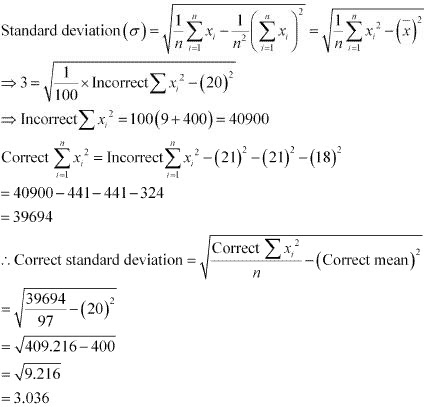# Class 11 Maths NCERT Solutions for Chapter 15 Statistics Miscellaneous Exercise### Statistics Miscellaneous Exercise Solutions

1. The mean and variance of eight observations are 9 and 9.25, respectively. If six of the observations are 6, 7, 10, 12, 12 and 13, find the remaining two observations.

Solution

Let the remaining two observations be x and y.
Therefore, the observations are 6, 7, 10, 12, 12, 13, xy.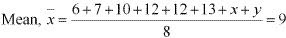⇒ 60 + x+  y = 72
⇒ x + y = 12  ...(1)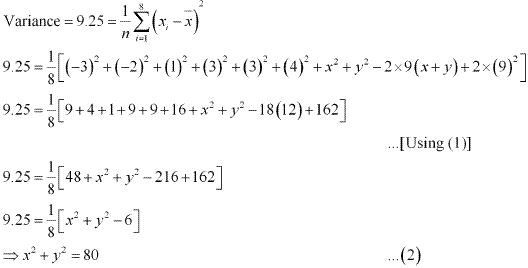From (1), we obtain
x2 + y2 + 2xy = 144 …(3)
From (2) and (3), we obtain
2xy = 64 … (4)
Subtracting (4) from (2), we obtain
x2 + y– 2xy = 80 – 64 = 16
⇒ x – y = ± 4 …(5)
Therefore, from (1) and (5), we obtain
x = 8 and y = 4, when x – y = 4
x = 4 and y = 8, when x – y = –4
Thus, the remaining observations are 4 and 8.

2. The mean and variance of 7 observations are 8 and 16, respectively. If five of the observations are 2, 4, 10, 12 and 14. Find the remaining two observations.

Solution

Let the observations be x1, x2, x3, x4, x5, and x6.
It is given that mean is 8 and standard deviation is 4.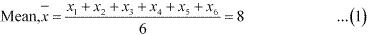If each observation is multiplied by 3 and the resulting observations are yi, then yi = 3x, i.e., xi = (1/3) yi, for i = 1 to 6.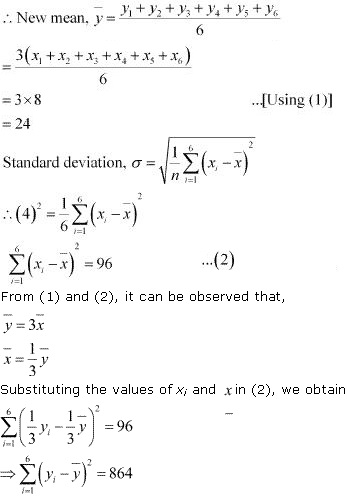Therefore, variance of new observations = (1/6) × 864 = 144
Hence, the standard deviation of new observations is √144 = 12

4. Given that x is the mean and σ2 is the variance of observations x1x2 … xn. Prove that the mean and variance of the observations ax1ax2ax3 …axare ax and a2 σ2, respectively (a ≠ 0).
Solution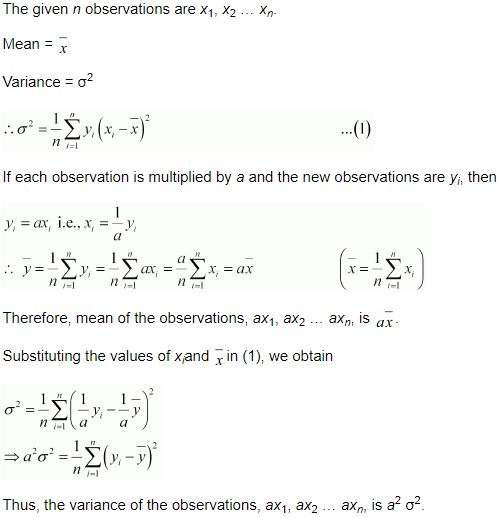5. The mean and standard deviation of 20 observations are found to be 10 and 2, respectively. On rechecking, it was found that an observation 8 was incorrect. Calculate the correct mean and standard deviation in each of the following cases:
(i) If wrong item is omitted.
(ii) If it is replaced by 12.
Solution
(i) Number of observations (n) = 20
Incorrect mean = 10
Incorrect standard deviation = 2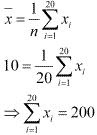That is, incorrect sum of observations = 200
Correct sum of observations  = 200 - 8 = 192
Correct mean = (Correct sum )/19 = 192/19 = 10.1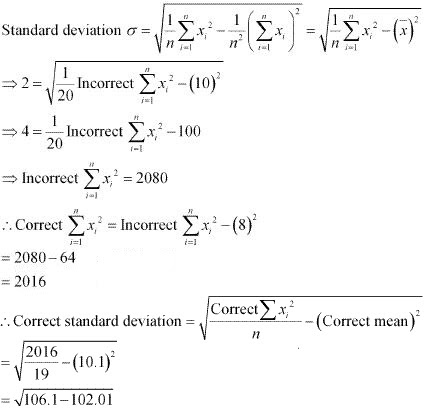= √4.09
= 2.02

(ii) When 8 is replaced by 12,
Incorrect sum of observations = 200
Correct sum of observations = 200 - 8 + 12 = 204
∴ Correct mean = (Correct sum)/20 = 204/20 = 10.2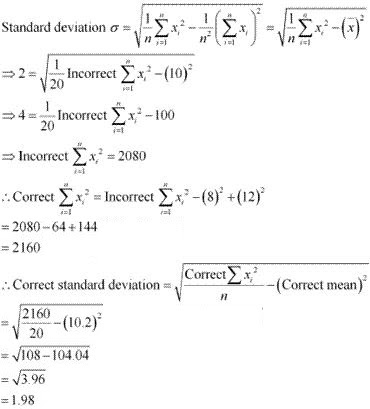6. The mean and standard deviation of marks obtained by 50 students of a class in three subjects, Mathematics, Physics and Chemistry are given below:
 Subject Mathematics Physics Chemistry Mean 42 32 40.9 Standard deviation 12 15 20

Which of the three subjects shows the highest variability in marks and which shows the lowest?

Solution

Standard deviation of Mathematics = 12
Standard deviation of Physics = 15
Standard deviation of Chemistry = 20
The coefficient of variation (C.V.) is given by (Standard deviation )/Mean  × 100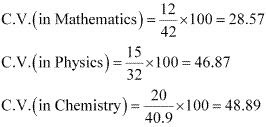The subject with greater C.V. is more variable than others.
Therefore, the highest variability in marks is in Chemistry and the lowest variability in marks is in Mathematics.

7. The mean and standard deviation of a group of 100 observations were found to be 20 and 3, respectively. Later on it was found that three observations were incorrect, which were recorded as 21, 21 and 18. Find the mean and standard deviation if the incorrect observations are omitted.

Solution

Number of observations (n) = 100
Incorrect mean x = 20

Incorrect standard deviation (σ) = 3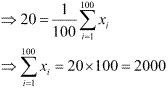Incorrect sum of observations  = 2000
Correct sum of observations  = 2000 - 21 - 21 - 18 = 2000 - 60 = 1940
∴ Correct mean = (correct sum)/(100 - 3) = 1940/97 = 20